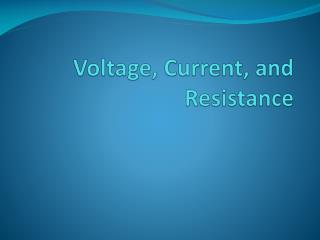DownloadDownload PresentationVoltage, Current, and Resistance

# Voltage, Current, and Resistance

Télécharger la présentation## Voltage, Current, and Resistance

- - - - - - - - - - - - - - - - - - - - - - - - - - - E N D - - - - - - - - - - - - - - - - - - - - - - - - - - -
##### Presentation Transcript

1. Voltage, Current, and Resistance

2. Objective of Lecture • Discuss resistivity and the three categories of materials • Chapter 2.1 • Show the mathematical relationships between charge, current, voltage, and energy. • Chapter 2.2-2.4 • Define resistance and conductance and how to determine the value of a resistor by a color code. • Chapter 2.5 • Explain the differences between electron flow, used in the text, and current flow, used in the field of electrical and computer engineering. • Chapter 2.4 and 2.6

3. Resistivity, r • Resistivity is a material property • Dependent on the number of free or mobile charges (usually electrons) in the material. • In a metal, this is the number of electrons from the outer shell that are ionized and become part of the ‘sea of electrons’ • Dependent on the mobility of the charges • Mobility is related to the velocity of the charges. • It is a function of the material, the frequency and magnitude of the voltage applied to make the charges move, and temperature.

4. Resistivity of Common Materials at Room Temperature (300K)

5. Electrical Components • Resistors, capacitors, and inductors are electrical components as opposed to diodes, transistors, and other components fabricated using semiconductor materials, which are electronic components. • These electrical components are fabricated using metals, which can allow electrons to flow easily through them, and insulators, which allow almost no electrons to flow.

6. Charge • Electrical property of atomic particles • Electrons are negatively charged • Protons are positivity charged • The absolute value of the charge on an electron is 1.6x10-19 C • The symbol used is Q or q • Uppercase is used to denote a steady-state or constant value • Lowercase is used to denote an instantaneous value or time-varying quantity

7. Voltage (Potential Difference) • The electromotive force (emf) that causes charge to move. • 1 Volt = 1 Joule/1 Coulomb

8. DC vs. AC • DC (or dc) is the acronym for direct current. • The current remains constant with time. • Uppercase variables are used when calculating dc values. • AC (or ac) is the acronym for alternating current. • Specifically, AC current varies sinusoidally with time and the average value of the current over one period of the sinusoid is zero. • Lowercase variables are used when calculating ac values. • Other time-varying currents exist, but there isn’t an acronym defined for them.

9. Ideal Voltage Sources • Independent voltage source outputs a voltage, either dc or time varying, to the circuit no matter how much current is required.

10. Types of DC Voltage Sources • Batteries • Electrochemical potential developed between an anode and cathode • Fuel cells • Solar cells (Photovoltaics) • DC generators • Electromagnetic induction to produce voltage • Thermocouples • Piezoelectric devices

11. Current • The flow of charge through a cross-sectional area as a function of time or the time rate of change of charge • Symbol used is I or i

12. Current Sources • An ideal current source outputs a dc or ac current to the circuit no matter how much voltage is required. • There are no ideal current sources as all known current sources are unable to generate an unlimited force (voltage) that is needed to deliver a constant current to a circuit.

13. Remember that: • Current can flow in and out of an independent voltage source, but the polarity of the voltage is determined by the voltage source. • There is always a voltage drop across the independent current source and the direction of positive current is determined by the current source.

14. Resistance, R • Resistance takes into account the physical dimensions of the material where: L is the length along which the carriers are moving A is the cross sectional area that the free charges move through.

15. Conductance, G • Conductance is the reciprocal of resistance G = R-1 = i/v Unit for conductance is S (siemens) or (mhos) G = As/L where s is conductivity, which is the inverse of resistivity, r

16. Types of Resistors • Fixed resistors • Carbon composite resistors are most common • Metal film surface mount chip resistors • Wire wound resistors • Variable resistors • Trim potentiometers • Thermistors • Photoresistors • Strain gauges

17. Electron Flow and Current • Electrical and computer engineers use a convention for the direction of current that was defined by Ben Franklin. • We define conventional current as the time rate of change of positive charges, which is the opposite direction from the flow of electrons. • The text defines current as the flow of electrons, which is typically the definition used in disciplines such as Physics. • I will use the time rate of change of positive charges during this semester, which means either that the direction of positive current in my examples will be the opposite direction shown in the book or the magnitude of the currents will have opposite signs.

18. Summary • Resistivity is a fundamental material property while the material properties and the geometry of the component determine its resistance. • Conductance (conductivity) is the inverse of resistance (resistivity). • Voltage, current, and charge are related mathematically. • Conventional current, not electron current, will be used in this course.